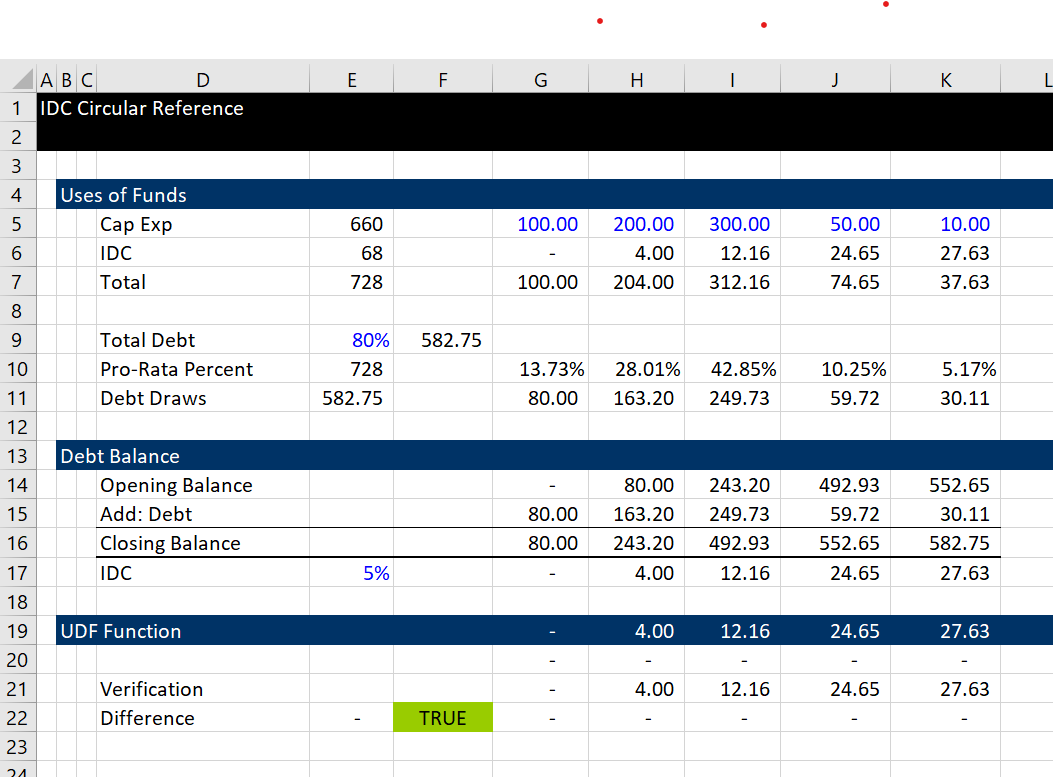# Pre-Course Exercise and Applying Parallel Concept to Your Models – Fundamental Cases

This page includes selected relatively simple exercise of applying the user defined function to resolve circular references. The user defined function method allows you to avoid problems that occur with both the iteration method (no goal seek; unstable models; slow models) and the copy and paste method (lose transparency; stop model execution; difficulty with data tables).  I start with very simple examples that only have a few inputs and do not have do loops.  Then I move to more complex cases with loops and reading in more variables. Each of the cases involves first understanding how the financial model works and then working through the excel model with equations.  All of the cases involving making a model parallel to your excel model. I have included the code on this page and also included the sample files.

## Case 1: Simple Funding without Loop

For the first case I have set up a very simple sources and uses of funds model with fees on debt. The fees are computed on debt and are included in the uses of funds. In the example you can contrast different methods for solving circular references.

## Case 5: Interest During Construction with Loop

This exercise demonstrates how to address the classic circular reference loop in a project finance model.  The screenshot below demonstrates how the debt size comes from the total cost which in turn comes from the interest during construction. Then, the pro-rata percent for determining how much debt is issue comes from a ratio of the total expenditures including IDC.  To solve this circular reference problem this time you need a loop.  With a loop you need to re-set things to zero and be more carefule with the order of things. The UDF function is in line 19 and is an array function.  Arguments for the UDF function include the debt to capital percent, the interest rate and the series of capital expenditures.The circular reference is illustrated below.  The total debt drives the IDC which drives the pro-rata percentage.  The pro-rata percentage level then drives the IDC which in turn drives the pro-rata percent.  When the blue arrows go back and forth like they do in the diagram below, you probably need a loop to solve the problem.  You also need to put the output of the UDF into an array variable rather than a single variable. The file that has this example is attached to the button below.The code below illustrates computation of a UDF in the loop.  I have put comments in the loop.  Note that you do not need to put anything in the UDF to say that it is an array output.  Note also that the total debt is computed separately from the debt balance.

```Option Base 1 ' Use option base 1

Function idc_result(int_rate, pct_debt, cap_exp) ' The output is an array variable

Dim idc(10) As Double ' Put arrays on variables you need to remember

For iter = 1 To 20 ' This is arbitrary; it should be modified to check convergence

project_cost = 0 ' Reset the project cost before the loop

For i = 1 To cap_exp.Count
project_cost = project_cost + cap_exp(i) + idc(i) ' Cap Exp is an array variable because of the way it is read in
Next i

debt_balance = 0
debt = project_cost * pct_debt ' You need the project cost to get the debt

For i = 1 To cap_exp.Count
uses = cap_exp(i) + idc(i)
pro_rata = uses / project_cost ' Compute the pro-rata
draws = pro_rata * debt ' Draws from pro-rata

idc(i) = debt_balance * int_rate

debt_balance = debt_balance + draws
Next i

Next iter

idc_result = idc ' The result does not have to be an array

End Function

```